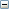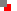﻿ ListsLength(LS:string|list): number;
 Function If LS is a string expression, then LENGTH returns the number of characters in the string. If LS is a list, then LENGTH returns the number of elements in the list.Table example: Variable data See also LIST_STRINGS function.List_Strings(L:list;I:number):string;
 Function LIST_STRINGS function returns the element of list L specified by index number I as a string.Table example: Variable data See also LENGTH, LIST_NUMBERS functions.List_Add(L:list;S:string):number;
 Function LIST_ADD adds a string element S to the list L then returns the element index number.List_Clear(L:list);
 Procedure LIST_CLEAR removes all elements in list L.List_Numbers(L:list;I:number):number
 Function LIST_NUMBERS returns element I of list L as a number. If the list element cannot be interpreted as a number then LIST_NUMBERS returns -1. See also LENGTH, LIST_STRINGS functions.List_IndexOf(L:list;SearchFor:string;[CaseBlind:boolean=true;[StartIndex:number=0]]):number;
 Function LIST_INDEXOF returns the index of a specified string SEARCHFOR in list L. If the specified string is not found then LIST_INDEXOF returns -1. The function defaults to case-blind string matching and searches from the first element of the list.l:=['alpha','Beta','gamma']; writeln(list_indexof(l,'beta')); // returns 1 writeln(list_indexof(l,'beta',false)); // returns -1 See also LIST_STRINGS, LIST_NUMBERS functions.